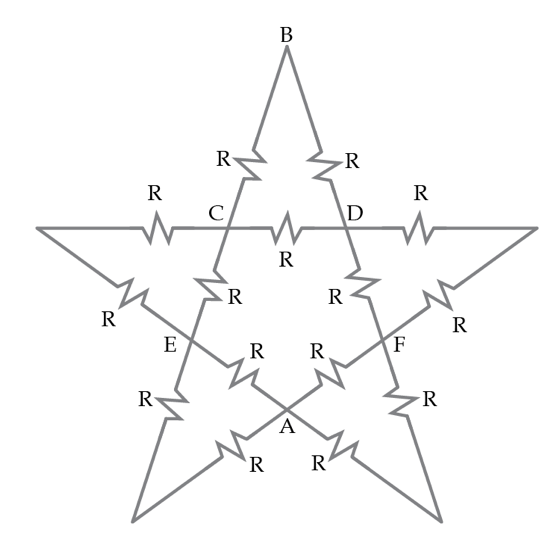# We are all made of stars

Consider a five-pointed star made of resistors as shown below. Each resistor has resistance $R=6~\Omega$. Find the equivalent resistance in Ohms between the points A and B.Details and assumptions

Note: Points ABCDE are labeled for easy reference if you need to use some combination of them in a solution.

×